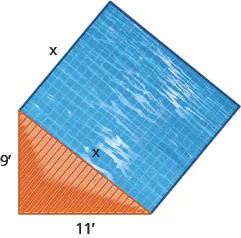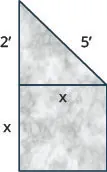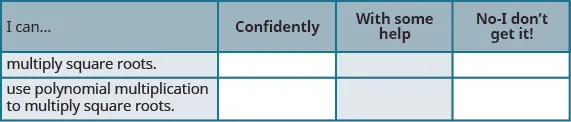Elementary Algebra

# 9.4Multiply Square Roots

Elementary Algebra9.4 Multiply Square Roots

### Learning Objectives

By the end of this section, you will be able to:

• Multiply square roots
• Use polynomial multiplication to multiply square roots

### Be Prepared 9.4

Before you get started, take this readiness quiz.

1. Simplify: $(3u)(8v)(3u)(8v)$.
If you missed this problem, review Example 6.26.
2. Simplify: $6(12−7n)6(12−7n)$.
If you missed this problem, review Example 6.28.
3. Simplify: $(2+a)(4−a)(2+a)(4−a)$.
If you missed this problem, review Example 6.39.

### Multiply Square Roots

We have used the Product Property of Square Roots to simplify square roots by removing the perfect square factors. The Product Property of Square Roots says

$ab=a·bab=a·b$

We can use the Product Property of Square Roots ‘in reverse’ to multiply square roots.

$a·b=aba·b=ab$

Remember, we assume all variables are greater than or equal to zero.

We will rewrite the Product Property of Square Roots so we see both ways together.

### Product Property of Square Roots

If a, b are nonnegative real numbers, then

$ab=a·banda·b=abab=a·banda·b=ab$

So we can multiply $3·53·5$ in this way:

$3·53·5153·53·515$

Sometimes the product gives us a perfect square:

$2·82·81642·82·8164$

Even when the product is not a perfect square, we must look for perfect-square factors and simplify the radical whenever possible.

Multiplying radicals with coefficients is much like multiplying variables with coefficients. To multiply $4x·3y4x·3y$ we multiply the coefficients together and then the variables. The result is $12xy12xy$. Keep this in mind as you do these examples.

### Example 9.44

Simplify: $2·62·6$ $(43)(212)(43)(212)$.

### Try It 9.87

Simplify: $3·63·6$ $(26)(312)(26)(312)$.

### Try It 9.88

Simplify: $5·105·10$ $(63)(56)(63)(56)$.

### Example 9.45

Simplify: $(62)(310)(62)(310)$.

### Try It 9.89

Simplify: $(32)(230)(32)(230)$.

### Try It 9.90

Simplify: $(33)(36)(33)(36)$.

When we have to multiply square roots, we first find the product and then remove any perfect square factors.

### Example 9.46

Simplify: $(8x3)(3x)(8x3)(3x)$ $(20y2)(5y3)(20y2)(5y3)$.

### Try It 9.91

Simplify: $(6x3)(3x)(6x3)(3x)$ $(2y3)(50y2)(2y3)(50y2)$.

### Try It 9.92

Simplify: $(6x5)(2x)(6x5)(2x)$ $(12y2)(3y5)(12y2)(3y5)$.

### Example 9.47

Simplify: $(106p3)(318p)(106p3)(318p)$.

### Try It 9.93

Simplify: $(62x2)(845x4)(62x2)(845x4)$.

### Try It 9.94

Simplify: $(26y4)(1230y)(26y4)(1230y)$.

### Example 9.48

Simplify: $(2)2(2)2$ $(−11)2(−11)2$.

### Try It 9.95

Simplify: $(12)2(12)2$ $(−15)2(−15)2$.

### Try It 9.96

Simplify: $(16)2(16)2$ $(−20)2(−20)2$.

The results of the previous example lead us to this property.

### Squaring a Square Root

If a is a nonnegative real number, then

$(a)2=a(a)2=a$

By realizing that squaring and taking a square root are ‘opposite’ operations, we can simplify $(2)2(2)2$ and get 2 right away. When we multiply the two like square roots in part (a) of the next example, it is the same as squaring.

### Example 9.49

Simplify: $(23)(83)(23)(83)$ $(36)2(36)2$.

### Try It 9.97

Simplify: $(611)(511)(611)(511)$ $(58)2(58)2$.

### Try It 9.98

Simplify: $(37)(107)(37)(107)$ $(−46)2(−46)2$.

### Use Polynomial Multiplication to Multiply Square Roots

In the next few examples, we will use the Distributive Property to multiply expressions with square roots.

We will first distribute and then simplify the square roots when possible.

### Example 9.50

Simplify: $3(5−2)3(5−2)$ $2(4−10)2(4−10)$.

### Try It 9.99

Simplify: $2(3−5)2(3−5)$ $3(2−18)3(2−18)$.

### Try It 9.100

Simplify: $6(2+6)6(2+6)$ $7(1+14)7(1+14)$.

### Example 9.51

Simplify: $5(7+25)5(7+25)$ $6(2+18)6(2+18)$.

### Try It 9.101

Simplify: $6(1+36)6(1+36)$ $12(3+24)12(3+24)$.

### Try It 9.102

Simplify: $8(2−58)8(2−58)$ $14(2+42)14(2+42)$.

When we worked with polynomials, we multiplied binomials by binomials. Remember, this gave us four products before we combined any like terms. To be sure to get all four products, we organized our work—usually by the FOIL method.

### Example 9.52

Simplify: $(2+3)(4−3)(2+3)(4−3)$.

### Try It 9.103

Simplify: $(1+6)(3−6)(1+6)(3−6)$.

### Try It 9.104

Simplify: $(4−10)(2+10)(4−10)(2+10)$.

### Example 9.53

Simplify: $(3−27)(4−27)(3−27)(4−27)$.

### Try It 9.105

Simplify: $(6−37)(3+47)(6−37)(3+47)$.

### Try It 9.106

Simplify: $(2−311)(4−11)(2−311)(4−11)$.

### Example 9.54

Simplify: $(32−5)(2+45)(32−5)(2+45)$.

### Try It 9.107

Simplify: $(53−7)(3+27)(53−7)(3+27)$.

### Try It 9.108

Simplify: $(6−38)(26+8)(6−38)(26+8)$

### Example 9.55

Simplify: $(4−2x)(1+3x)(4−2x)(1+3x)$.

### Try It 9.109

Simplify: $(6−5m)(2+3m)(6−5m)(2+3m)$.

### Try It 9.110

Simplify: $(10+3n)(1−5n)(10+3n)(1−5n)$.

Note that some special products made our work easier when we multiplied binomials earlier. This is true when we multiply square roots, too. The special product formulas we used are shown below.

### Special Product Formulas

$Binomial SquaresProduct of Conjugates(a+b)2=a2+2ab+b2(a−b)(a+b)=a2−b2(a−b)2=a2−2ab+b2Binomial SquaresProduct of Conjugates(a+b)2=a2+2ab+b2(a−b)(a+b)=a2−b2(a−b)2=a2−2ab+b2$

We will use the special product formulas in the next few examples. We will start with the Binomial Squares formula.

### Example 9.56

Simplify: $(2+3)2(2+3)2$ $(4−25)2(4−25)2$.

### Try It 9.111

Simplify: $(10+2)2(10+2)2$ $(1+36)2(1+36)2$.

### Try It 9.112

Simplify: $(6−5)2(6−5)2$ $(9−210)2(9−210)2$.

### Example 9.57

Simplify: $(1+3x)2(1+3x)2$.

### Try It 9.113

Simplify: $(2+5m)2(2+5m)2$.

### Try It 9.114

Simplify: $(3−4n)2(3−4n)2$.

In the next two examples, we will find the product of conjugates.

### Example 9.58

Simplify: $(4−2)(4+2)(4−2)(4+2)$.

### Try It 9.115

Simplify: $(2−3)(2+3)(2−3)(2+3)$.

### Try It 9.116

Simplify: $(1+5)(1−5)(1+5)(1−5)$.

### Example 9.59

Simplify: $(5−23)(5+23)(5−23)(5+23)$.

### Try It 9.117

Simplify: $(3−25)(3+25)(3−25)(3+25)$.

### Try It 9.118

Simplify: $(4+57)(4−57)(4+57)(4−57)$.

### Media

Access these online resources for additional instruction and practice with multiplying square roots.

### Section 9.4 Exercises

#### Practice Makes Perfect

Multiply Square Roots

In the following exercises, simplify.

233.

$2·82·8$
$(33)(218)(33)(218)$

234.

$6·66·6$
$(32)(232)(32)(232)$

235.

$7·147·14$
$(48)(58)(48)(58)$

236.

$6·126·12$
$(25)(210)(25)(210)$

237.

$( 5 2 ) ( 3 6 ) ( 5 2 ) ( 3 6 )$

238.

$( 2 3 ) ( 4 6 ) ( 2 3 ) ( 4 6 )$

239.

$( −2 3 ) ( 3 18 ) ( −2 3 ) ( 3 18 )$

240.

$( −4 5 ) ( 5 10 ) ( −4 5 ) ( 5 10 )$

241.

$( 5 6 ) ( − 12 ) ( 5 6 ) ( − 12 )$

242.

$( 6 2 ) ( − 10 ) ( 6 2 ) ( − 10 )$

243.

$( −2 7 ) ( −2 14 ) ( −2 7 ) ( −2 14 )$

244.

$( −2 11 ) ( −4 22 ) ( −2 11 ) ( −4 22 )$

245.

$(15y)(5y3)(15y)(5y3)$
$(2n2)(18n3)(2n2)(18n3)$

246.

$(14x3)(7x3)(14x3)(7x3)$
$(3q2)(48q3)(3q2)(48q3)$

247.

$(16y2)(8y4)(16y2)(8y4)$
$(11s6)(11s)(11s6)(11s)$

248.

$(8x3)(3x)(8x3)(3x)$
$(7r)(7r8)(7r)(7r8)$

249.

$( 2 5 b 3 ) ( 4 15 b ) ( 2 5 b 3 ) ( 4 15 b )$

250.

$( 3 8 c 5 ) ( 2 6 c 3 ) ( 3 8 c 5 ) ( 2 6 c 3 )$

251.

$( 6 3 d 3 ) ( 4 12 d 5 ) ( 6 3 d 3 ) ( 4 12 d 5 )$

252.

$( 2 5 b 3 ) ( 4 15 b ) ( 2 5 b 3 ) ( 4 15 b )$

253.

$( 6 3 d 3 ) ( 4 12 d 5 ) ( 6 3 d 3 ) ( 4 12 d 5 )$

254.

$( −2 7 z 3 ) ( 3 14 z 8 ) ( −2 7 z 3 ) ( 3 14 z 8 )$

255.

$( 4 2 k 5 ) ( −3 32 k 6 ) ( 4 2 k 5 ) ( −3 32 k 6 )$

256.

$(7)2(7)2$
$(−15)2(−15)2$

257.

$(11)2(11)2$
$(−21)2(−21)2$

258.

$(19)2(19)2$
$(−5)2(−5)2$

259.

$(23)2(23)2$
$(−3)2(−3)2$

260.

$(411)(−311)(411)(−311)$
$(53)2(53)2$

261.

$(213)(−913)(213)(−913)$
$(65)2(65)2$

262.

$(−312)(−26)(−312)(−26)$
$(−410)2(−410)2$

263.

$(−75)(−310)(−75)(−310)$
$(−214)2(−214)2$

Use Polynomial Multiplication to Multiply Square Roots

In the following exercises, simplify.

264.

$3(4−3)3(4−3)$
$2(4−6)2(4−6)$

265.

$4(6−11)4(6−11)$
$2(5−12)2(5−12)$

266.

$5(3−7)5(3−7)$
$3(4−15)3(4−15)$

267.

$7(−2−11)7(−2−11)$
$7(6−14)7(6−14)$

268.

$7(5+27)7(5+27)$
$5(10+18)5(10+18)$

269.

$11(8+411)11(8+411)$
$3(12+27)3(12+27)$

270.

$11(−3+411)11(−3+411)$
$3(15−18)3(15−18)$

271.

$2(−5+92)2(−5+92)$
$7(3−21)7(3−21)$

272.

$( 8 + 3 ) ( 2 − 3 ) ( 8 + 3 ) ( 2 − 3 )$

273.

$( 7 + 3 ) ( 9 − 3 ) ( 7 + 3 ) ( 9 − 3 )$

274.

$( 8 − 2 ) ( 3 + 2 ) ( 8 − 2 ) ( 3 + 2 )$

275.

$( 9 − 2 ) ( 6 + 2 ) ( 9 − 2 ) ( 6 + 2 )$

276.

$( 3 − 7 ) ( 5 − 7 ) ( 3 − 7 ) ( 5 − 7 )$

277.

$( 5 − 7 ) ( 4 − 7 ) ( 5 − 7 ) ( 4 − 7 )$

278.

$( 1 + 3 10 ) ( 5 − 2 10 ) ( 1 + 3 10 ) ( 5 − 2 10 )$

279.

$( 7 − 2 5 ) ( 4 + 9 5 ) ( 7 − 2 5 ) ( 4 + 9 5 )$

280.

$( 3 + 10 ) ( 3 + 2 10 ) ( 3 + 10 ) ( 3 + 2 10 )$

281.

$( 11 + 5 ) ( 11 + 6 5 ) ( 11 + 5 ) ( 11 + 6 5 )$

282.

$( 2 7 − 5 11 ) ( 4 7 + 9 11 ) ( 2 7 − 5 11 ) ( 4 7 + 9 11 )$

283.

$( 4 6 + 7 13 ) ( 8 6 − 3 13 ) ( 4 6 + 7 13 ) ( 8 6 − 3 13 )$

284.

$( 5 − u ) ( 3 + u ) ( 5 − u ) ( 3 + u )$

285.

$( 9 − w ) ( 2 + w ) ( 9 − w ) ( 2 + w )$

286.

$( 7 + 2 m ) ( 4 + 9 m ) ( 7 + 2 m ) ( 4 + 9 m )$

287.

$( 6 + 5 n ) ( 11 + 3 n ) ( 6 + 5 n ) ( 11 + 3 n )$

288.

$(3+5)2(3+5)2$
$(2−53)2(2−53)2$

289.

$(4+11)2(4+11)2$
$(3−25)2(3−25)2$

290.

$(9−6)2(9−6)2$
$(10+37)2(10+37)2$

291.

$(5−10)2(5−10)2$
$(8+32)2(8+32)2$

292.

$( 3 − 5 ) ( 3 + 5 ) ( 3 − 5 ) ( 3 + 5 )$

293.

$( 10 − 3 ) ( 10 + 3 ) ( 10 − 3 ) ( 10 + 3 )$

294.

$( 4 + 2 ) ( 4 − 2 ) ( 4 + 2 ) ( 4 − 2 )$

295.

$( 7 + 10 ) ( 7 − 10 ) ( 7 + 10 ) ( 7 − 10 )$

296.

$( 4 + 9 3 ) ( 4 − 9 3 ) ( 4 + 9 3 ) ( 4 − 9 3 )$

297.

$( 1 + 8 2 ) ( 1 − 8 2 ) ( 1 + 8 2 ) ( 1 − 8 2 )$

298.

$( 12 − 5 5 ) ( 12 + 5 5 ) ( 12 − 5 5 ) ( 12 + 5 5 )$

299.

$( 9 − 4 3 ) ( 9 + 4 3 ) ( 9 − 4 3 ) ( 9 + 4 3 )$

Mixed Practice

In the following exercises, simplify.

300.

$3 · 21 3 · 21$

301.

$( 4 6 ) ( − 18 ) ( 4 6 ) ( − 18 )$

302.

$( −5 + 7 ) ( 6 + 21 ) ( −5 + 7 ) ( 6 + 21 )$

303.

$( −5 7 ) ( 6 21 ) ( −5 7 ) ( 6 21 )$

304.

$( −4 2 ) ( 2 18 ) ( −4 2 ) ( 2 18 )$

305.

$( 35 y 3 ) ( 7 y 3 ) ( 35 y 3 ) ( 7 y 3 )$

306.

$( 4 12 x 5 ) ( 2 6 x 3 ) ( 4 12 x 5 ) ( 2 6 x 3 )$

307.

$( 29 ) 2 ( 29 ) 2$

308.

$( −4 17 ) ( −3 17 ) ( −4 17 ) ( −3 17 )$

309.

$( −4 + 17 ) ( −3 + 17 ) ( −4 + 17 ) ( −3 + 17 )$

#### Everyday Math

310.

A landscaper wants to put a square reflecting pool next to a triangular deck, as shown below. The triangular deck is a right triangle, with legs of length 9 feet and 11 feet, and the pool will be adjacent to the hypotenuse.

1. Use the Pythagorean Theorem to find the length of a side of the pool. Round your answer to the nearest tenth of a foot.
2. Find the exact area of the pool.311.

An artist wants to make a small monument in the shape of a square base topped by a right triangle, as shown below. The square base will be adjacent to one leg of the triangle. The other leg of the triangle will measure 2 feet and the hypotenuse will be 5 feet.

1. Use the Pythagorean Theorem to find the length of a side of the square base. Round your answer to the nearest tenth of a foot.2. Find the exact area of the face of the square base.
312.

A square garden will be made with a stone border on one edge. If only $3+103+10$ feet of stone are available, simplify $(3+10)2(3+10)2$ to determine the area of the largest such garden.

313.

A garden will be made so as to contain two square sections, one section with side length $5+65+6$ yards and one section with side length $2+32+3$ yards. Simplify $(5+6)(2+3)(5+6)(2+3)$ to determine the total area of the garden.

314.

Suppose a third section will be added to the garden in the previous exercise. The third section is to have a width of $432432$ feet. Write an expression that gives the total area of the garden.

#### Writing Exercises

315.
1. Explain why $(−n)2(−n)2$ is always positive, for $n≥0n≥0$.
2. Explain why $(−n)2(−n)2$ is always negative, for $n≥0n≥0$.
316.

Use the binomial square pattern to simplify $(3+2)2(3+2)2$. Explain all your steps.

#### Self Check

After completing the exercises, use this checklist to evaluate your mastery of the objectives of this section.On a scale of 1–10, how would you rate your mastery of this section in light of your responses on the checklist? How can you improve this?

Order a print copy

As an Amazon Associate we earn from qualifying purchases.